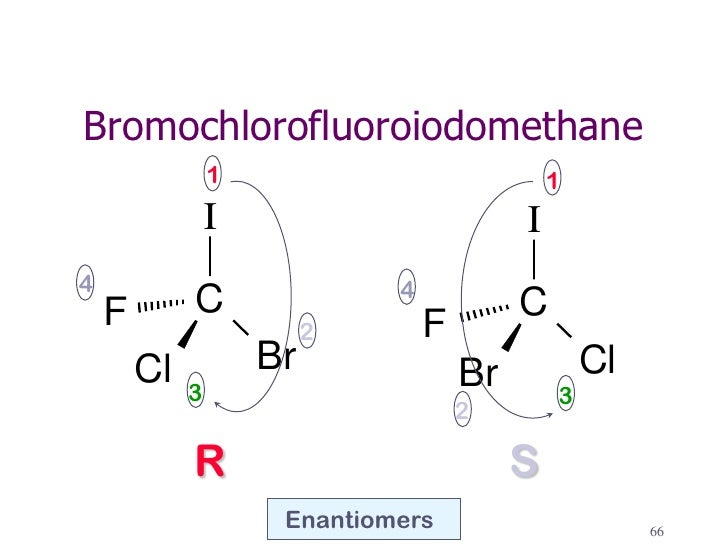# How can I show the R-configuration of the molecule bromochlorofluoroiodomethane?

Dec 24, 2014

Here's how I do it.

Step 1. Draw a C atom with a wedge, a dash, and two solid bonds.Step 2. Add F, Cl, Br, and I to the bonds in any order.

We have a 50:50 chance of getting it right. Here's one possibility.Step 3. Assign priorities to the groups.

I = 1; Br = 2; Cl = 3; F = 4.

Step 4. Assign stereochemistry

F has the lowest priority. In this drawing, it is more convenient to view the molecule from above.

Then I → Br→ Cl = 1 → 2 → 3 goes in a counterclockwise direction ($S$).

But we are viewing the molecule with our eye closest to the low-priority group.

So this is really ($R$)-bromochlorofluoroiodomethane.

If we had gotten it wrong, we would have interchanged any two of the groups.

For example, in the diagram below, interchanging the Cl and Br atoms converts the $R$ isomer to the $S$ isomer.